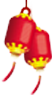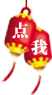# Java零基础教程-运算符-赋值类运算符

• 网盘提取码：k9w1
• 适合对象：Java学员、Java爱好者、开发人员
• qq号：2158476996零基础免费学

## 下载介绍运算符 运算规则 范例 结果 = 赋值 int a=2 2 += 加后赋值 int a=2，a+=2 4 -= 减后赋值 int a=2，a-=2 0 *= 乘后赋值 int a=2，a*=2 4 /= 整除后赋值 int a=2，a/=2 1 %= 取模后赋值 int a=2，a%=2 0

注意：诸如+=这样形式的赋值运算符，会将结果自动强转成等号左边的数据类型。

## 学员感言